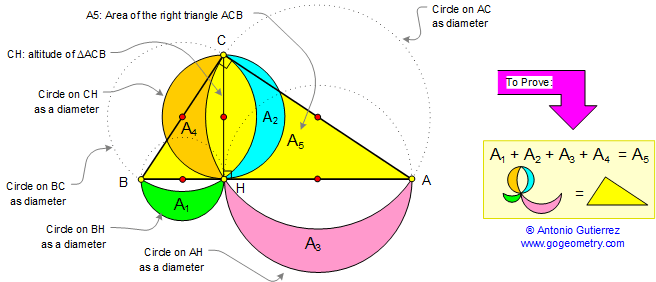# Math: Geometry: Lune of Hippocrates 4: Circle Areas and Right Triangle

In the figure below, prove that the area of a right triangle ACB of altitude CH is equal to the sum of the areas of the lunes A1, A2, A3, and A4.Hippocrates of Chios (c.470 BC - c.410 BC) was a Greek mathematician who worked on the classical problems of squaring the circle and duplicating the cube.

Squaring the circle or the quadrature of the circle  is one of the three great problems of Classical Geometry. It is the challenge to construct a square with the same area as a given circle by using only a finite number of steps with compass and straightedge.

See also: Hippocrates 1, Hippocrates 2, Hippocrates 3, Squaring the Circle, Pythagoras and Circle Area, Similar shapes.

Home | Geometry | Pythagoras Index | Right Triangle Formulas | Right Triangle Area | Lune Index | Semicircle | Circular Segment | Email | by Antonio Gutierrez